nextnano.com  GUI: nextnanomat  Tool: nextnano++  Tool: nextnano³  Tool: nextnano.NEGF  Download | Search | Copyright | News | Publications  * password protected nextnano³ software1D piezo 

# nextnano3 - Tutorial

## Piezoelectric field in InAs/GaAs QWs grown along the  orientation

Author: Stefan Birner

If you want to obtain the input files that are used within this tutorial, please contact stefan.birner@nextnano.de.
``` -> 1DInAsQW_onGaAs111_piezo_11nm_nn3.in        / *_nnp.in - ```input file for the nextnano3 and nextnano++ software```  -> 1DInGaAsQW_onGaAs111_piezo_2ndOrder_nn3.in  / *_nnp.in - ```input file for the nextnano3 and nextnano++ software```  -> 1DInGaAsQW_onGaAs111_piezo_nn3.in           / *_nnp.in - ```input file for the nextnano3 and nextnano++ software```  ```

## Piezoelectric field in InAs/GaAs QWs grown along the  orientation

In this tutorial we study the effect of the piezoelectric field of an InAs QW embedded between GaAs barrries.

Sign convention of the piezoelectric constant in zinc blende materials

According to T. Ikeda [Fundamentals of piezoelectricity, Oxford Science Publications, 1st ed., p. 53 (1990)] the sign of the piezoelectric constant is taken as positive if a positive charge is induced in the positive direction of the axis under a positive (tensile) stress.
In nextnano³ we define as the positive  direction the one from cation (i.e. metal ion, e.g. Ga in GaAs) to anion (e.g. As in GaAs), i.e. parallel to the Ga-As bond. In this case the piezoelectric constant can be both positive (AlP, InP) and negative (AlAs, InAs, GaAs). Note that this is the same definition as for wurtzite where the positive  direction is defined from cation to anion and also the same definition that is used by the wafer manufacturers.
This definition has also been used in the article Piezoelectricity by R. M. Martin, Physical Review B 5, 1607 (1972).
cation: positively charged ion, e.g. Ga3+
anion:  negatively charged ion, e.g. As3`-`

This tutorial is based on the following paper:

`[Bester]`
Importance of Second-Order Piezoelectric Effects in Zinc-Blende Semiconductors
G. Bester, X. Wu, D. Vanderbilt, A. Zunger
Physical Review Letters 96, 187602 (2006)

We use a 11 nm InxGa1-xAs quantum well embedded between 14 nm GaAs barriers on each side.
We employ periodic boundary conditions to the Poisson equation which is equivalent to a 11 nm InGaAs / 28 nm GaAs superlattice.
The heterostructure is strained pseudomorphically with respect to GaAs substrate, i.e. GaAs is unstrained, InGaAs is compressively strained.

## InAs quantum well between GaAs

``` -> 1DInAsQW_onGaAs111_piezo_11nm_nn3.in     %AlloyContent = 1.0  ! InAs , alloy content In(x)Ga(1-x)As (DisplayUnit:)     %QW_width     = 11.0 ! quantum well width                  (DisplayUnit:nm)  ```

### Strain

For more information on strain, please have a look at this tutorial: Strain and displacement tensors along different growth directions

For a compressively strained InAs layer grown along the  direction on GaAs, we obtain the following strain tensor components:

• As GaAs is unstrained, all strain tensor components are zero in GaAs.
• In InAs, the strain tensors components with respect to the crystal coordinate system are as follows:
epsxx = epsyy = epszz = `-`0.03219 (i.e. negative)
epsxy = epsxz = epsyz =   0.03533 (i.e. positive)
• The trace of the strain tensor is negative, i.e. the hydrostatic strain epshydro = Delta V / V = `-`0.09656 which is negative as it should be for a compressed crystal.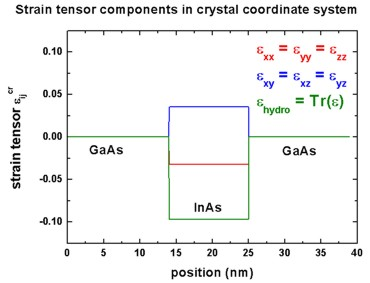### Polarization and piezoelectric charge density

``` -> 1DInAsQW_onGaAs111_piezo_11nm_nn3.in     %QW_width = 11.0   ! quantum well width (DisplayUnit:nm)     %InAs_e14 = -0.045 ! 1st order piezoelectric coefficient e14 (InAs), experimental value (DisplayUnit:C/m^2) (ListOfValues:-0.045,-0.115)```

In a zinc blende crystal, the polarization with respect to the crystal coordinate system is defined as  P = e14  (2 epsyz , 2 epsxz , 2 epsxy).
Consequently, in GaAs the polarization is zero because the strain tensor is zero.
In compressively strained InAs, the polarization P is negative if e14 is negative (along the  direction) and because the off-diagonal strain tensor components are positive.

In this example, we use the following experimentally determined piezoelectric constants for bulk InAs:

• InAs:   e14 = `-`0.045 C/m2

We compare this value to the value as calculated by` [Bester]`.

• InAs:   e14 = `-`0.115 C/m2     (However, we neglect second-order terms.)

The piezoelectric charge density is definded as: rhopiezo = ``` -``` div P

P (GaAs) = 0
P (InAs)   = negative
P (GaAs) = 0

`==> `left   interface: rhopiezo = `-` d/dz Pz = `-` [Pz(InAs)  `-` Pz (GaAs)] / (Delta_z) = positive value
`==> `right interface: rhopiezo = `-` d/dz Pz = `-` [Pz(GaAs) `-` Pz (InAs)]  / (Delta_z) = negative value

Consequently, for a compressively strained InAs QW, a positive piezo charge density arises on the left interface, and a negative piezo charge density at the right interface.

The piezoelectric interface charge densities are calculated to be:
Filename:``` densities/interface_densitiesD.txt ```For InAs: e14 = `-`0.045 C/m2, the results are:```   Interface number 1 at position 14.0000000000000 nm        ```(left interface)```   piezoelectric charge:  5.506839895904100E-003 C/m^2       ```(positive)```   piezoelectric charge:  3.43709934129674 1E12  |e| / cm^2   Interface number 2 at position 25.0000000000001 nm        ```(right interface)```   piezoelectric charge: -5.506839895904100E-003 C/m^2       ```(negative)```   piezoelectric charge: -3.43709934129674 1E12  |e| / cm^2 ```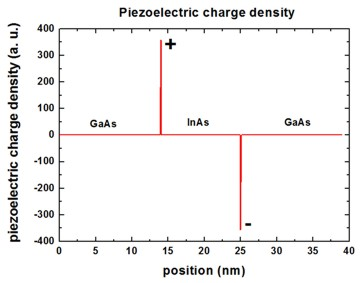### Piezoelectric potential

```    %InAs_e14 = -0.045 ! 1st order piezoelectric coefficient e14 (InAs), experimental value (DisplayUnit:C/m^2) (ListOfValues:-0.045,-0.115)```

The piezoelectric potential phipiezo is the solution of the Poisson equation, i.e. the electrostatic potential (or electric potential) which is potential energy per unit charge.
Note: The piezoelectric potential energy function Vpiezo which enters the Hamiltonian is defined as: Vpiezo = `-` e phipiezo
In the following figure we plot phipiezo (and not Vpiezo which would have the opposite sign) in units of [eV] where "e" is the charge quantum |e|.The piezoelectric charges are localized at the interfaces leading to a linear potential.

### Piezoelectric potential including second-order terms for piezoelectric polarization

``` -> 1DInGaAsQW_onGaAs111_piezo_11nm_2ndOrder_nn3.in     %Include2ndOrderPiezo = yes     %AlloyContent = 1.00   ! InAs          , alloy content In(x)Ga(1-x)As (DisplayUnit:)(ListOfValues:0.20,1.00)     %AlloyContent = 0.20   ! In0.20Ga0.80As, alloy content In(x)Ga(1-x)As (DisplayUnit:)(ListOfValues:0.20,1.00)  ```

To include this effect, we have to switch on the 2nd order effect for the piezoelectric polarization in``` \$numeric-control```.
```    piezo-second-order = no    ! (default)                       = yes   !```

The following figure shows the same as above but this time including the second-order terms B114, B124, B156 for the piezoelectric polarization.
Note that the maximum value of our potential is at 0.55 eV whereas``` [Bester] ```finds 0.85 eV for InAs.
Also included is the result for In0.2Ga0.8As (including second-order terms, dotted line).
We found that at an Indium concentration of about x = ~0.60 the sign change occurs (`[Bester]`: x = ~ 0.35).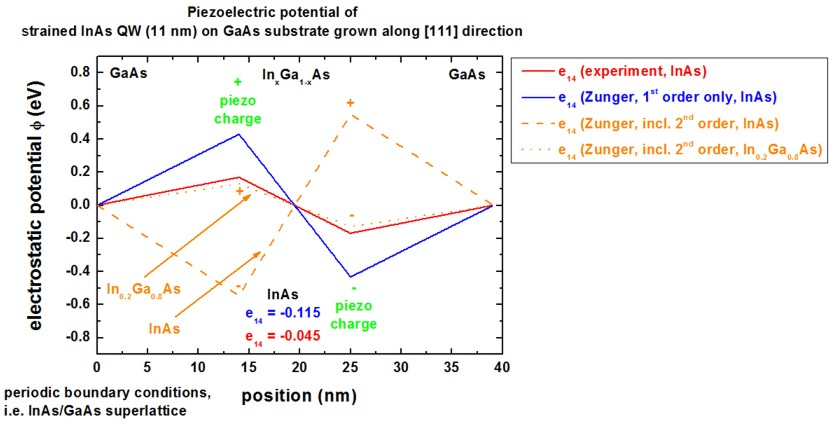### Piezoelectric field

```    %Include2ndOrderPiezo = no     %AlloyContent = 1.00   ! InAs, alloy content In(x)Ga(1-x)As (DisplayUnit:)     %InAs_e14     = -0.045 ! 1st order piezoelectric coefficient e14 (InAs), experimental value (DisplayUnit:C/m^2) (ListOfValues:-0.045,-0.115)```

The piezoelectric field is defined as F = `-` grad phipiezo.
Inside the InAs quantum well, the field has a positive value, which is equivalent to a negative slope along the  direction for the piezoelectric potential.
Note: [Bester] reports a negative value for the field although they have the same slope.
The piezoelectric field is found to be ~ -1000 kV/cm for the case when second-order terms are included whereas` [Bester] `finds ~ +1500 kV/cm.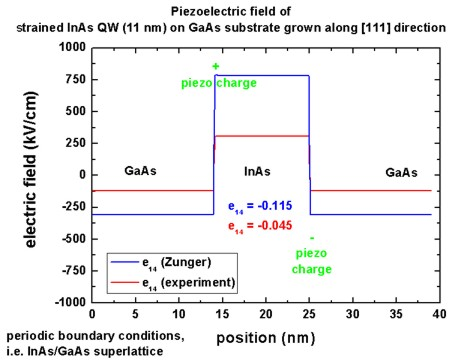### Conduction band edge

The following figure shows the resulting conduction band edge.
It is defined as Ec = Ec,0 `-` |e| phipiezo = Ec,0 + Vpiezo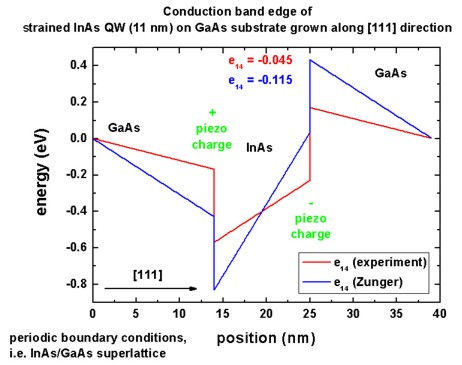## InGaAs quantum well between GaAs (realistic indium concentrations for quantum wells)

``` -> 1DInGaAsQW_onGaAs111_piezo_nn3.in     ```Here, we have to modify the alloy content, and the quantum well width.```     %AlloyContent = 0.21   ! In0.21Ga0.79As, alloy content In(x)Ga(1-x)As (DisplayUnit:)(ListOfValues:0.17,0.21)     %AlloyContent = 0.17   ! In0.17Ga0.83As, alloy content In(x)Ga(1-x)As (DisplayUnit:)(ListOfValues:0.17,0.21)     %QW_width     =  8.7   ! quantum well width (DisplayUnit:nm)(ListOfValues:8.7,10.0)     %QW_width     = 10.0   ! quantum well width (DisplayUnit:nm)(ListOfValues:8.7,10.0)     %GaAs_e14     = -0.160 ! 1st order piezoelectric coefficient e14 (GaAs), experimental value S. Gironcoli et al., PRL 62(24), 2853 (1989) (DisplayUnit:C/m^2)     %InAs_e14     = -0.045 ! 1st order piezoelectric coefficient e14 (InAs), experimental value (DisplayUnit:C/m^2)  ```

Now we calculate three different InxGa1-xAs quantum wells with different well widths and indium alloy contents and compare our results to experimental data.

 quantum well material well width piezoelectric field (experiment) piezoelectric field (nextnano³) In0.17Ga0.83As 8.7 nm 129 kV/cm +`-` 12 kV/cm [Cho, 2001] 196 kV/cm In0.17Ga0.83As 10 nm 137 kV/cm +`-` 6 kV/cm [Sanchez, 2002] 188 kV/cm In0.21Ga0.79As 10 nm 121 kV/cm +`-` 5 kV/cm [Sanchez, 2002] 223 kV/cm

For these calculations, we used the experimental values for the piezoelectric constants:

InAs:   e14 = `-`0.045 C/m2
GaAs: e14 = `-`0.160 C/m2

The experimental trends

• "larger well width means larger field" and
• "more indium means less field"

cannot be reproduced.

We also note that our results for the piezoelectric field have the opposite sign compared to the calculations of` [Bester]`.
A different convention for the  direction (cation to anion vs. anion to cation) could be a possible explanation for this.

• Please help us to improve our tutorial. Send comments to``` support [at] nextnano.com```.